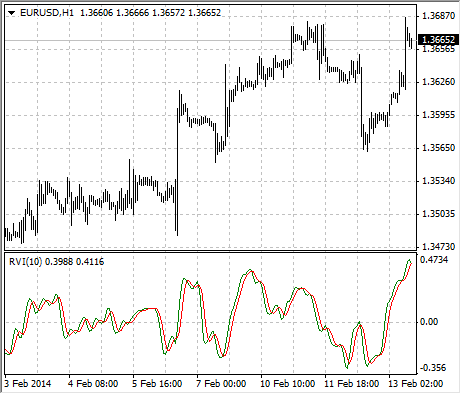Relative Vigor Index

# Relative Vigor Index

The main point of Relative Vigor Index Technical Indicator (RVI) is that on the bull market the closing price is, as a rule, higher, than the opening price. It is the other way round on the bear market. So the idea behind Relative Vigor Index is that the vigor, or energy, of the move is thus established by where the prices end up at the close. To normalize the index to the daily trading range, divide the change of price by the maximum range of prices for the day. To make a more smooth calculation, one uses Simple Moving Average. 10 is the best period. To avoid probable ambiguity one needs to construct a signal line, which is a 4-period symmetrically weighted moving average of Relative Vigor Index values. The concurrence of lines serves as a signal to buy or to sell.## Calculation

RVI = (CLOSE-OPEN)/(HIGH-LOW)

Where:

OPEN – is the opening price;
HIGH – is the maximum price;
LOW – is the minimum price;
CLOSE – is the closing price.